### Day 21 - Chain Rule - 02.02.16

 Update Unit 2 TestFriday, 02.12.16Calculus Equations/Theorems Find the derivative of the following: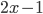2x2-10none of the aboveFind the derivative of the following: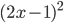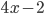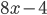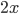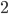none of the aboveFind the derivative of the following: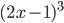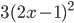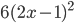none of the aboveReviewPre-calculusSlopeEquation of a LineSecant Line vs. Tangent Line (video)DerivativeHow can the slope of a tangent line be found? Equation of a Tangent Line (video)Tangent Line GraphDerivative (video) (checkpoints)Basic Differentiation Rules (checkpoints)Constant Rule (video) (example)Power Rule (video) (example)Constant Multiple Rule (video) (example)Sum and Difference Rule (video)Derivative of Sine and Cosine FunctionsProduct Rule (video) and Quotient Rule (video) (checkpoints)How can derivatives of the product/quotient of functions be calculated?LessonChain Rule (video)Checkpoints Exit Ticket Posted on the board at the end of the block.HomeworkWNQ the following:Implicit Differentiation (video)Study! Help Request List Standard(s) APC.2Define and apply the properties of limits of functions.Limits will be evaluated graphically and algebraically.Includes:​limits of a constant​limits of a sum, product, and quotient​one-sided limits​limits at infinity, infinite limits, and non-existent limitsAPC.3Use limits to define continuity and determine where a function is continuous or discontinuous.Includes:​continuity in terms of limitscontinuity at a point and over a closed interval​application of the Intermediate Value Theorem and the Extreme Value Theorem​geometric understanding and interpretation of continuity and discontinuity